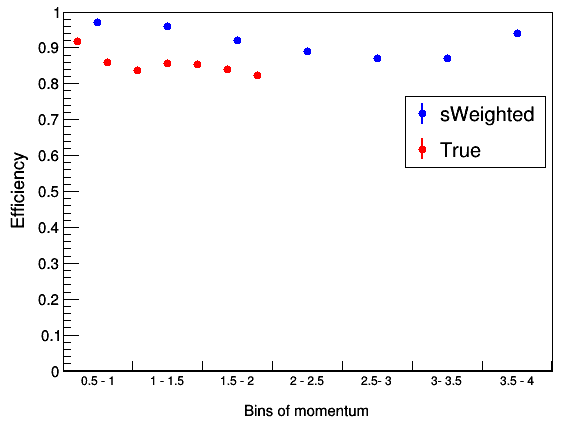# Plotting the data points from Tefficiency in customized bins

Dear Experts,

In this macro:efficiency.C (2.4 KB) and the root file: for_tefficiency_0.1.root (4.7 KB), I have plotted a simple graph, then plotted a graph with TEfficiency (with x-error bars removed). I am also able to draw them on the same axes using TMultigraph.

I also want to show the data points for both the graphs shown bin-by- bin (with bins on the x-axis) and so, I also created a 2D- Histogram with customised bin labels. Now, I am able to show the data points of the simple graph (blue) in customized bins but I am not able to do so for the data points generated from TEfficiency (red). This is how my plot currently looks like:.

Would you please help me solve this? I don’t want the red points to flock together. I want then one in each in bin just as the blue points are arranged.

Regards,
Sanjeeda

ROOT Version: 5.34
Platform: Ubuntu (16.04)
Compiler: Not Provided

The points of the 2 graphs have different x coordinates, so when you combine them in a multigraph, the points go to different bins in the painted histogram. You can substitute the x of the points of the second graph so that they are the same as the first one (or viceversa):

``````  for (int i = 0; i < gr2->GetN(); ++i) {
cout << gr2->GetPointX(i) << endl;
gr2->SetPointX(i,x[i]);
gr2->GetEXlow()[i] = 0;
gr2->GetEXhigh()[i] = 0;
}
``````

Instead of “`gr2->GetPointX(i)`”, use: `gr2->GetX()[i]`
Instead of “`gr2->SetPointX(i,x[i]);`”, use: `gr2->GetX()[i] = x[i]; // enforce same x-axis`

Dear @dastudillo and @Wile_E_Coyote,

Thank you very much. It works now.